Math Concepts

# Geometrical Lines

476 views

 1. Introduction 2. Types of lines 3. Worksheet 4. Summary 5. FAQS 6. External References

16th December 2020

## Introduction

In daily life, we use the word "line" very frequently and casually. But in Geometry's fantastic world, "lines" is considered a very core factor. The corners and lines collectively construct some basic shapes in Geometry.
For example, the rectangle is formed by putting together 4 lines at 90°.

So, what is a "line"?

The "line" is a mark or stroke connecting two dots. (usually to measure out the distance in those specific two dots).

Lines have a very descriptive and specific definition. A line is a one-dimensional object. It is made of a set of points, flowing infinitely towards both of its directions. It has a length but no width.

### Geometrical Lines-PDF

In this article, we will study Parallel lines,  Perpendicular lines,  Slope of line, etc. Also, we will solve some fascinating worksheets to explore the world of lines. Properties of parallel and perpendicular lines. Slope of a line. Here is a downloadable PDF to explore more.

## Types of lines

Equations can define geometrical lines and their properties. We can also determine the type of lines by seeing if they cross each other and which angle it is forming. The study of it throws light on types of lines.

### Parallel Lines

When two or more lines running alongside each other, without a change in distance between them, and without a meeting, they are called Parallel Lines.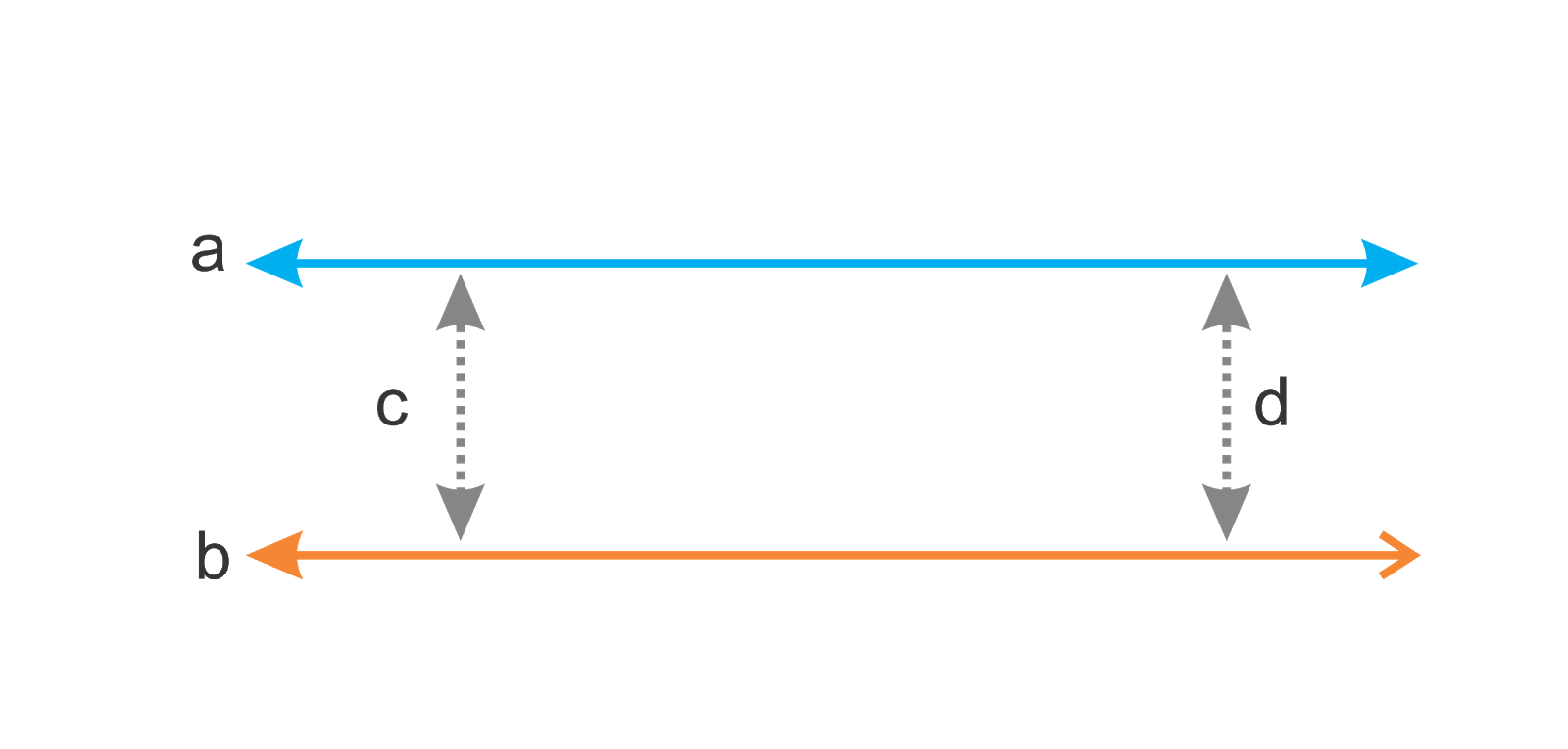In the above image, the spacing in lines “a” and “b” at any given point remains equal. If you see, the length of the line “c” and “d” (showing the distance between lines “a” and “b” at two different points selected) are equal. Hence, Line “a” and line “b” are parallel lines.A prevalent example of parallel lines is two sides of the ladder. The two sides of ladders have equal distance and never meet.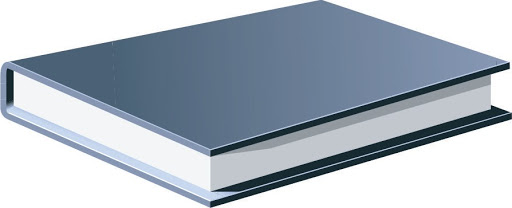You can have a look at your notebook. Both opposite sides of it have the same distance from the beginning till the end.
Here we will have a little diversion. You can see a notebook has a rectangle shape.
Any Rectangle is formed of equal lengths of parallel lines on its opposite sides.

Try to look around and find objects which are formed by Parallel lines. It will be a FUN activity.

### Perpendicular lines:

When two or more lines cross each other and form an angle of 900, they are perpendicular lines. 2]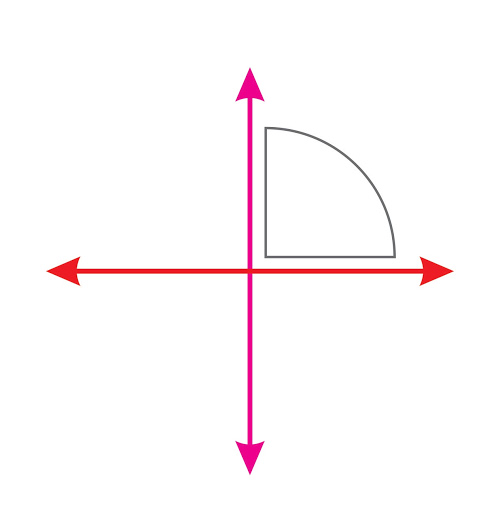A very commonly seen example of Perpendicular lines is a mathematical sign of “plus.”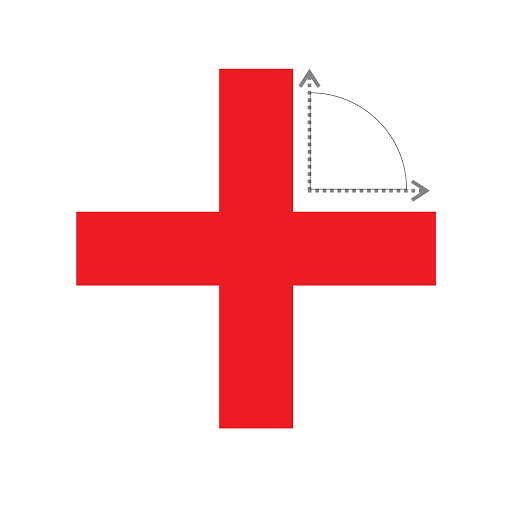The intersection of 2 lines forms the sign of  “+.” Both these lines cross each other and form a Right angle.

### Transversal lines:

The lines that intersect are called transversal lines. In the given image, the line “a” and line”b” cross each other and hence called the Transversal line.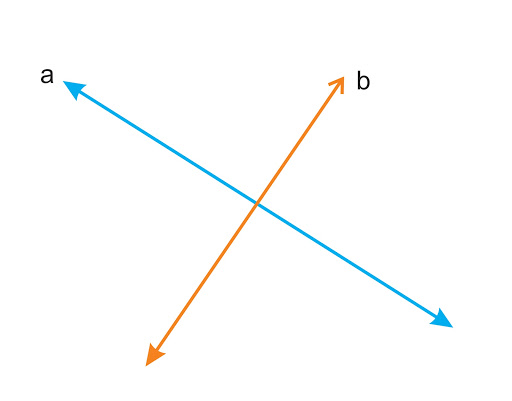We will solve some worksheets to know more about transversal lines.

### The Slope of the line:

Word slop indicates moving in a specific direction, either upward or opposite directions. In Geometry, the slope of a line is a number indicating the direction and steepness of a line. It is represented by “m”.

The slope of a line is very useful while reading graphs or charts. One can easily represent/study the behavior of the units on X-coordinates and Y-coordinates by calculating the slope of lines dotted on the graph.

Let's see How to calculate the slope of the line:-

Step 1 - Gets the difference between 2 points on Y-coordinates.

Step 2 - Gets the difference between the same 2 points (taken for X-coordinates) on X-coordinates.

Step 3 - Divide the difference between 2 same points on Y-coordinates by the difference in 2 points on X-coordinates.

 Example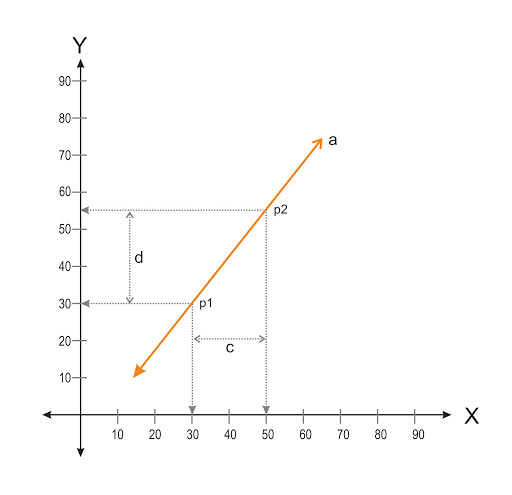In the above example
“d” is the difference between 2 points (p1 & p2) on Y-Coordinates. It is 55 - 30 = 25
“c” is the difference between 2 points  (p1 & p2) on X-Coordinates. It is 50 - 30 = 20

Formula to calculate the slope:
d /c

Hence, m = d/c

Slope (m) = 25/20 = 1.25

## Parallel Lines Worksheet

Parallel lines cut by transversal Worksheet

1 ]
In a given image, “a” & “b” are parallel lines, and “c” is a transversal line. What can be the value of “x” here? 5]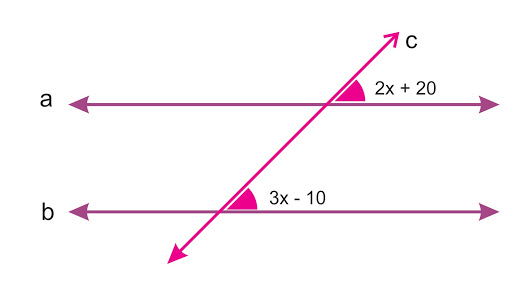As we learned, when transversal lines intersect parallel lines, the corresponding angles formed are equal.

So we can say,
2x + 20 = 3x - 10

X = 30
If we put value “x” in both angles, i.e.
2x + 20 = 2 × 30 + 20 = 80,
3x - 10 = 3 × 30 - 10 = 80

It proves both corresponding angles are equal.

2]
In a given image, “a” & “b” are parallel lin and “c” is a transversal line. What can be the value of “x” here?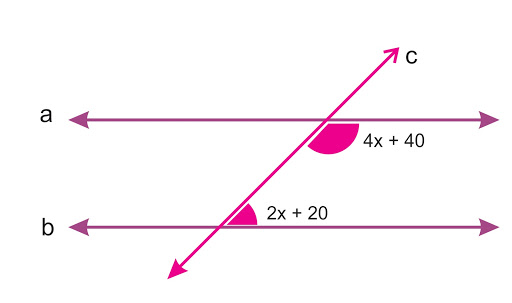As per properties of parallel lines, intersected by the Transversal line, Sum of consecutive interior angles is equal to 1800.

So, we can say that,
2x + 20  + 4x + 40 = 180
6x + 60 = 180
6x = 180-60 = 120
X = 20

Let’s see, the value of  consecutive interior angles
2x + 20 = 2 × 20 + 20 = 600
4x + 40 = 4 × 20 + 40 = 1200

## Summary

Defining Types of lines, and their equations with each other can be an exciting part of the study. When these lines are drawn to define specific information in a chart, it becomes. Self-explanatory.
Also, lines are a very important element in forming Geometric shapes.
Thus the practical implications and study of different types of lines are beneficial in many ways.

Cuemath, a student-friendly mathematics platform, conducts regular Online Live Classes for academics and skill-development and their Mental Math App, on both iOS and Android, is a one-stop solution for kids to develop multiple skills.

Know more about the Cuemath fee here, Cuemath Fee

## What are the properties of parallel lines?

When a transversal line intersects two parallel lines, 8 angles are formed, 4 interior angles, and 4 exterior angles. Interestingly, the alternate interior angles and alternate exterior angles are equal. 4]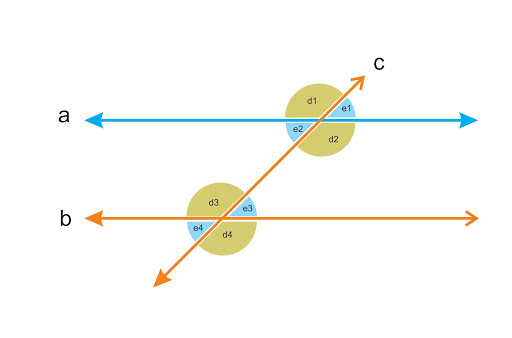Here we can see all alternate interior angles e1. e2, e3, and e4 are equal Also

• All exterior angles, d1, d2, d3, and d4, are equal.
• It never forms any angle & has equal slopes.
• They never intersect, no matter how long they are extended.

## Which geometrical shapes are formed by a set of perpendicular lines/parallel lines?

• Two sets of parallel lines on opposite sides form the shape of a Rectangle.
• A combination of four perpendicular lines in a specific way can form a square.
• Two sets of parallel lines in a specific way can form the shape of a parallelogram.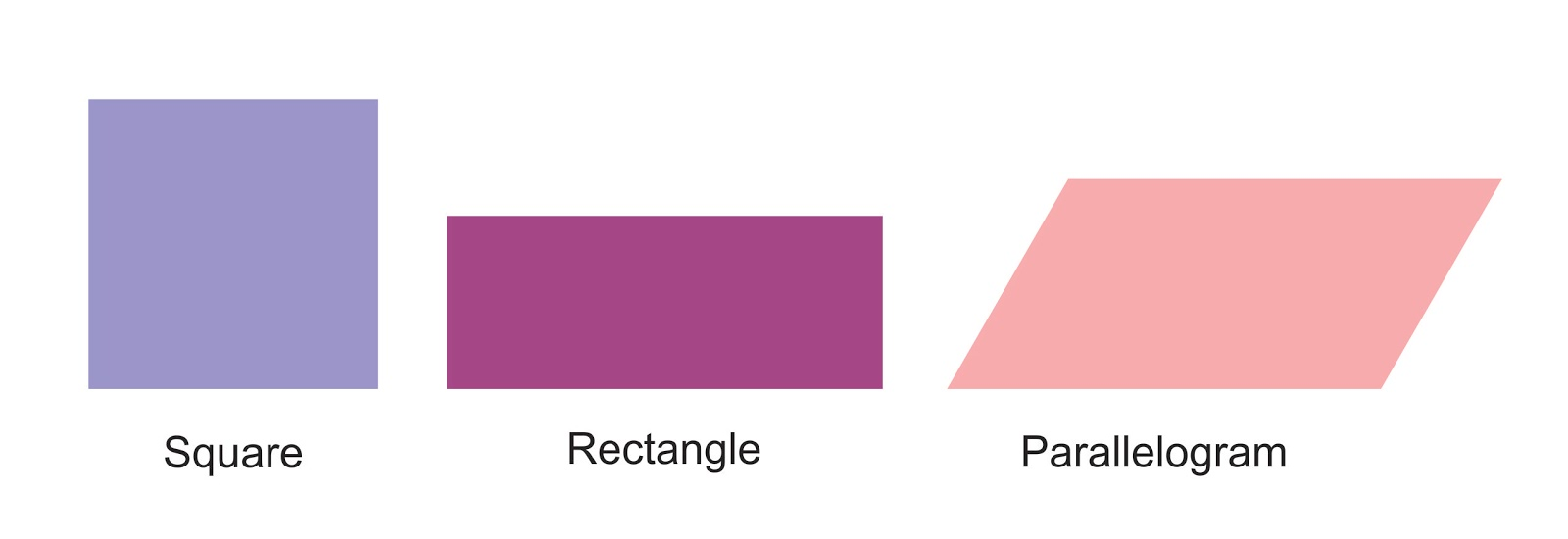## What are the properties of Perpendicular lines?

They form a right angle at the point at which they intersect each other.

## What are the properties of Transversal lines?

When the transversal line intersects two different lines, 8 angles are formed. These 8 angles are known as transversal angles and are called - Interior angles and Exterior angles. (The interesting part is when the line intersects two parallel lines, an angle formed by both alternate interior angle and alternate exterior angles are equal.)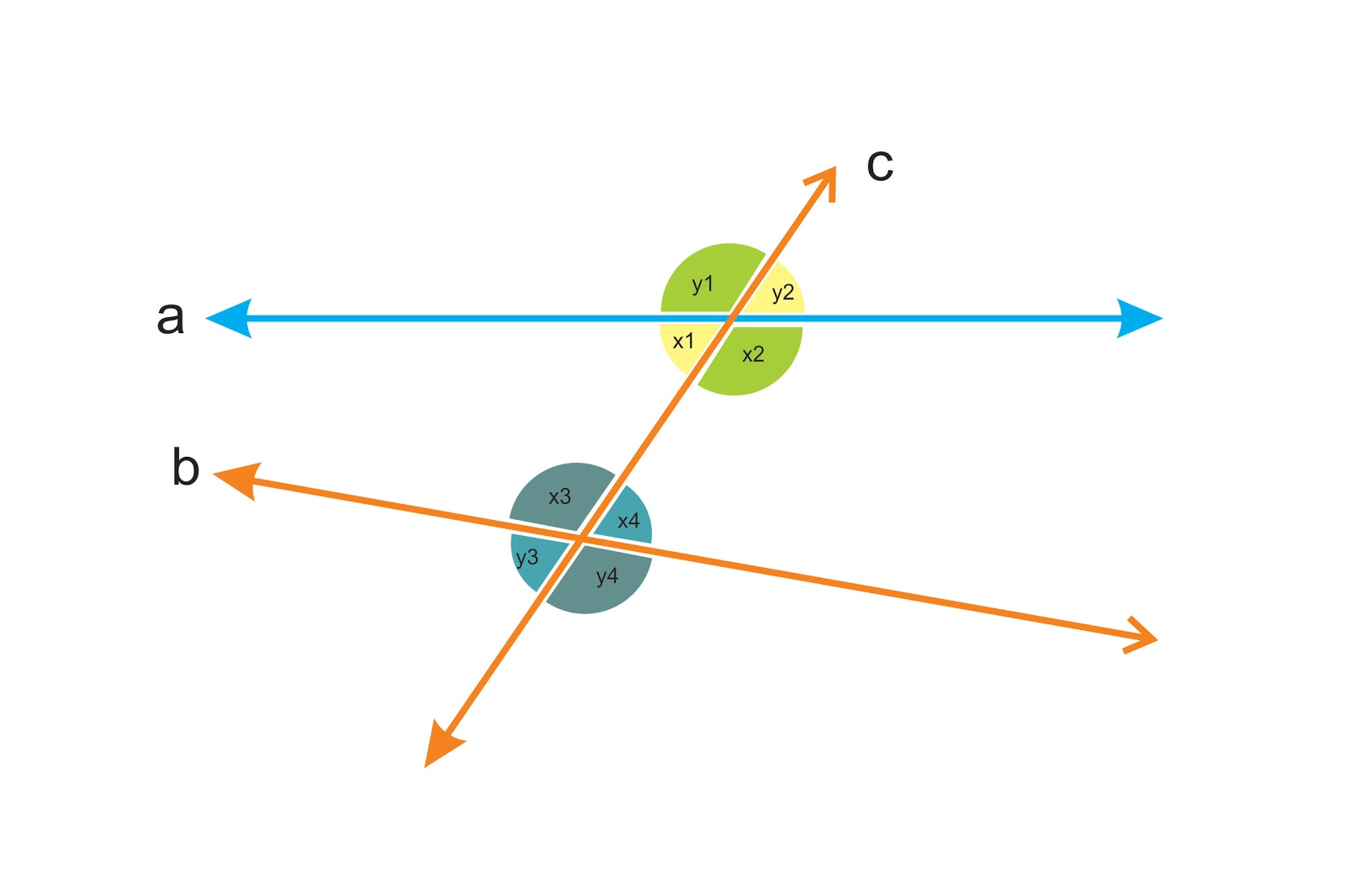In the above example, we can see, 8 angles are formed by one transversal line.
4 interior angle
es - x1, x2, x3 and x4
4 exterior angles - y1, y2, y3 and y4.

## How many types of slopes are there and their names?

There are 4 types of slopes. 2]
They are:
• Positive slope
• Negative Slope
• Zero Slope
• Undefined slope

## What is the relationship between the slopes of two parallel lines?

Two parallel lines always have the same slope.

## What is the relationship between the slopes of two perpendicular lines?

The slopes of two perpendicular lines are always the negative reciprocal of one another, i.e. if you multiply the value of the slope of the two lines you will always get -1.

Related Articles
GIVE YOUR CHILD THE CUEMATH EDGE
Access Personalised Math learning through interactive worksheets, gamified concepts and grade-wise courses